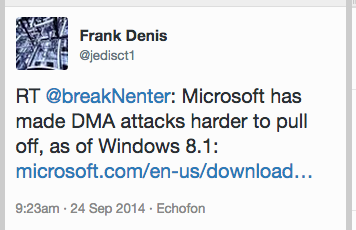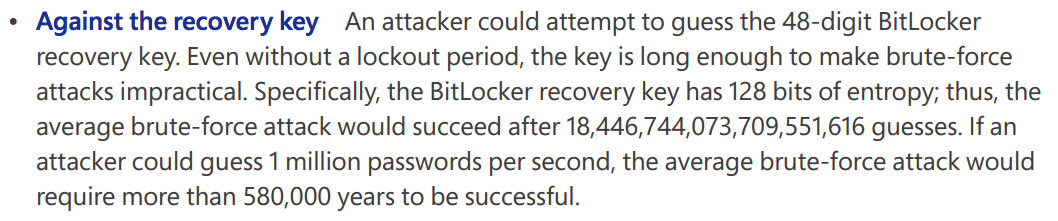# Seriously Bad Arithmetic by Microsoft

Today I saw this Tweet about Microsoft's mitigation of Direct Memory Access attacks:Here's the full link, which downloads a Microsoft PDF titled "Countermeasures: Protecting BitLocker-encrypted Devices from Attacks" dated January, 2014.

There's lots of good information in it, but a grievious arithmetical error. Here's the section I noticed:I immediately knew that was wrong--580,000 years is WAY too small, the correct time is "forever", or at least the age of the Universe, as explained here:

I've done a similar calculation recently, for my IPv6 Exhaustion Counter.

## Correct Arithmetic

Microsoft uses a 48-digit key. There are 10^48 possible keys.

A 128-bit key has 2^128 possible values, or 3.4 x 10^38. That's a lot smaller than 10^48, and may well be an error. But perhaps Microsoft is saying that ten(!) of the digits in the key are actually predictable from the others, for error correction or for some other reason.

However, Microsoft uses the number 1.8 x 10^19, which is 2^64, not 2^128. They may be thinking of hash collisions, not brute-force attacks.

How long will it take to crack a 128-bit key, guessing 1 million keys per second?

2^128 / 1 million seconds = 3.4 x 10^38 / 10^6 seconds = 3.4 x 10^32 seconds.

There are 3600 x 24 x 365 = 3.15 x 10^7 seconds in a year, so that's

3.4 x 10^32 / 3.15 x 10^7 years = 1.08 x 10^25 years.

In round numbers, that's 10,000,000,000,000,000,000,000,000 years.

The Universe is 13.8 x 10^9 years old (from Wikipedia, which is approximately 10,000,000,000 years.

So Microsoft's calculation is off by a staggering amount.

## Reality Check

This is all theoretical, and ignores the real problems of generating random keys using real hardware. As Dan Kaminsky explained at Defcon in 2012, real keys generated by computers tend to be predictable, causing the real keyspace to be potentially much smaller than the numbers would indicate:

I am a classic academic, with far more interest in abstract, ideal calculations than most hands-on engineers. But I am troubled by the fact that Microsoft published a document with an outrageous error like that in it nine months ago and no one has fixed it yet.

## Astrophysics

Another limit is the energy required to run a computer cracking a key. Here's Bruce Schneier explaining how it would take the entire output of the Sun for 32 years merely to run a counter through all possible 192-bit keys:

Posted 9-24-14 10:46 am by Sam Bowne